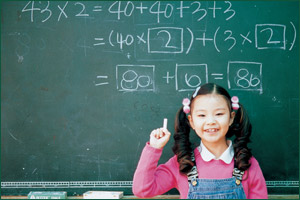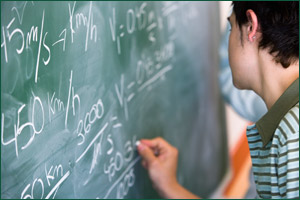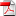# Mathematics Curriculum

## Elementary Mathematics Curriculum

Elementary mathematics students develop understanding of whole numbers, fractions, decimals, operations, measurement, and geometry. Students apply their understanding of these concepts to solve problems and communicate about mathematics in their world.## Secondary Mathematics Curriculum

Secondary mathematics students develop understanding of the number system, ratios and proportional relationships, expressions and equations, functions, algebra, geometry, and statistics and probability. Students apply their understanding of these concepts to solve problems and communicate about mathematics in their world.### Middle School

• Mathematics 6• Mathematics 7• Pre-Algebra GT• Mathematics 7• Mathematics 8• Algebra I G/T• Mathematics 8• Algebra I• Geometry GT